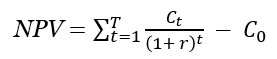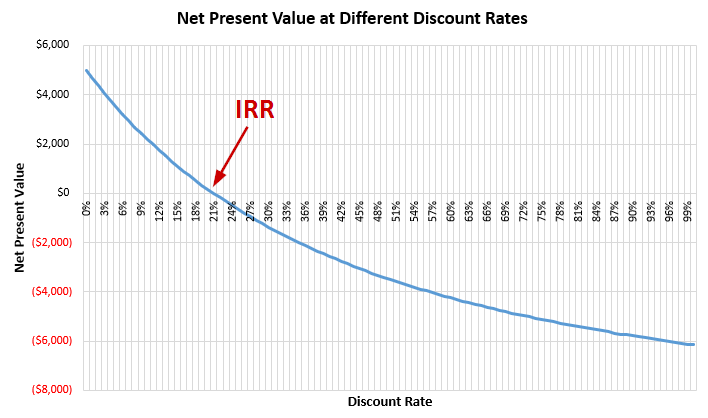# IRR Calculator

Use this online calculator to easily calculate the IRR (Internal Rate of Return) of any investment given the size of the investment and the cash flow per period. Also outputs gross return and net cash flow.

\$

Cash flows

\$
\$
\$
\$
\$
Share calculator:

Embed this tool:
get code

## Using the IRR calculator

Using the IRR calculation tool is straightforward: simply enter the initial investment (tool says dollars, but it can be in any currency like EUR, Swiss francs, etc.) then select the number of years of cash flow you want to analyze (could be any period, actually, but maximum 25 periods). Enter the cash flow for each period (past or future prediction).

Once ready, press "Calculate" and our Internal Rate of Return calculator will output:

• the Internal Rate of Return, a.k.a. discount rate
• the Gross Return in percentages
• the Net Cash Flow (Profit - Loss)

## What is Internal Rate of Return?

The Internal Rate of Return is the discount rate (interest rate) that makes the net present value (NPV) of all cash flows from a particular project equal to zero. It is also known as "economic rate of return" and "discounted cash flow rate of return", as well as a "hurdle rate" in the context of evaluation of potential investments. "Internal" in the name refers to the omission of external factors like capital cost, currency inflation, etc. By definition it gives more weight to earlier cash flows than to later cash flows reflecting the time preference of investors.

IRR is used to estimate the profitability of potential investments: the higher it is, the more desirable it is to undertake the project, while the lower it is, the more risky and overall undesirable. It is often used to rank prospective projects on a relatively even basis.

In general, it is best to combine IRR with other metrics such as NPV. It should be used with caution since comparing it across projects of widely different expected lengths can be misleading. Another issue to be taken into account is that IRR does not incorporate reinvestment rates which are more directly tied to the capital cost. Some propose the modified internal rate of return (MIRR) for cases when cost of capital is to be accounted for.

## IRR formula

If you wonder how to calculate the Internal Rate of Return by yourself or using an Excel spreadsheet, you would be surprised to discover that there is no analytical solution to the issue and the only way to calculate it is programmatically or by using tools such as our IRR calculator above. It is still based on the NPV formula:where r is the discount rate / interest rate and T is the number of cash flow periods with t denoting a specific period, C0 is the initial investment while Ct is the return during period t. One needs to replace NPV with zero and solve for r, for which there is no analytical solution since one can't single out r in one side of the equation. For this reason, our calculator performs a recursive search until it finds a value of r which results in an NPV close to zero.

Please, note that this formula only works for periodic payments, meaning that all cash flows need to happen at fixed time intervals, e.g. yearly, quarterly, monthly. For irregular (non-periodic) cash flows, please use our XIRR calculator.

## IRR calculation example

Let us examine the following investment scenario: a project requires an initial investment of \$10,000 and is expected to return \$15,000 in three years time with positive cash flows in each year of \$3,800, \$4,400, and \$6,800 respectively. What is the internal rate of return? Following from the definition of IRR we know that is the value of the discount rate at which the net present value becomes zero. One way to solve this problem is to graphically examine the relationship between the present value and the discount rate (a.k.a. cost of capital):From the graphical examination we can see that the NPV turns from positive to negative when the discount rate is between 20% and 21%. The process afterwards is of trying to successively approximate the internal rate of return by trying different discount rates in that range until we get the PV very close to zero. In this case the answer is 20.9%.

While the visual explanation in the above example is nice for getting a grasp of the concept of IRR, in practice the preferred approach is to use a software tool to do this automatically.

## Financial caution

This is a simple online calculator which is a good starting point in estimating the internal rate of return from an investment, but is by no means the end of such a process. You should always consult a qualified professional when making important financial decisions and long-term agreements, such as long-term bank deposits. Use the information provided by the software critically and at your own risk.

#### Cite this calculator & page

If you'd like to cite this online calculator resource and information as provided on the page, you can use the following citation:
Georgiev G.Z., "IRR Calculator", [online] Available at: https://www.gigacalculator.com/calculators/irr-calculator.php URL [Accessed Date: 27 Mar, 2023].